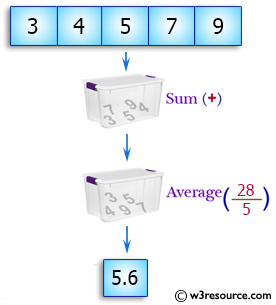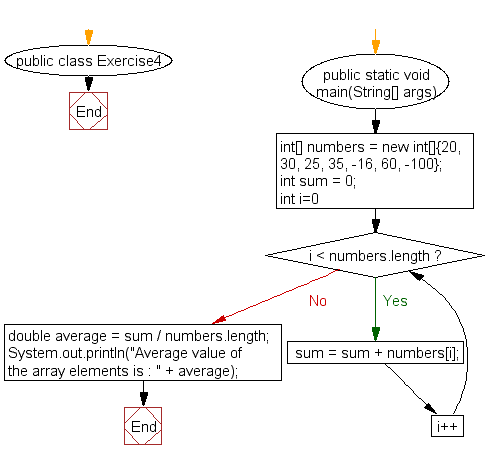﻿ Java exercises: Calculate the average value of array elements - w3resource# Java Array Exercises: Calculate the average value of array elements

## Java Array: Exercise-4 with Solution

Write a Java program to calculate the average value of array elements.

Pictorial Presentation:Sample Solution:

Java Code:

``````public class Exercise4 {
public static void main(String[] args) {

int[] numbers = new int[]{20, 30, 25, 35, -16, 60, -100};
//calculate sum of all array elements
int sum = 0;
for(int i=0; i < numbers.length ; i++)
sum = sum + numbers[i];
//calculate average value
double average = sum / numbers.length;
System.out.println("Average value of the array elements is : " + average);
}
}
```
```

Sample Output:

```Average value of the array elements is : 7.0
```

Flowchart:Visualize Java code execution (Python Tutor):

Java Code Editor:

Improve this sample solution and post your code through Disqus

What is the difficulty level of this exercise?

Test your Programming skills with w3resource's quiz.

﻿

## Java: Tips of the Day

countOccurrences

Counts the occurrences of a value in an array.

Use Arrays.stream().filter().count() to count total number of values that equals the specified value.

```public static long countOccurrences(int[] numbers, int value) {
return Arrays.stream(numbers)
.filter(number -> number == value)
.count();
}
```

Ref: https://bit.ly/3kCAgLb